Performance Review Score Calculation
• 25 Apr 2023
• Dark
Light

# Performance Review Score Calculation

• Dark
Light

Article Summary

As a result of the performance review, an employee can have two types of scores assigned: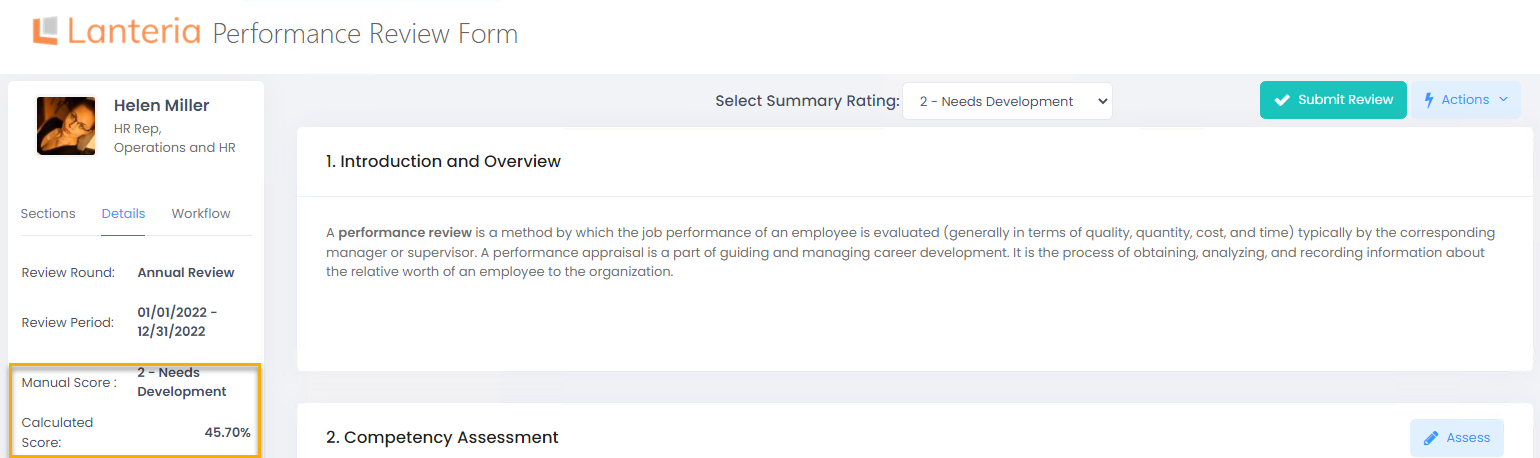The following video gives an overview of the score types and calculation process.

## Manual Score

Manual score or summary rating is assigned manually by the reviewer using the Select Summary Rating field of the performance review form. This is possible only on those review workflow steps that have the Assign Summary Rating check box selected (Settings > Settings and Configuration > Performance > Performance Reviews; click Edit next to Performance Review Workflows, click the workflow name and then the step name).

The system provides suggestion for the summary rating after the reviewer fills in the Summary Score section. This section type allows providing summary scores for the other sections included in the review form.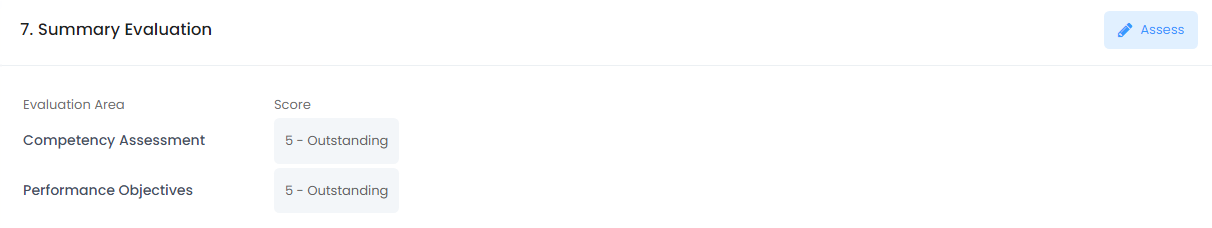An average score is displayed in the Select Summary Rating field, but can be overridden by the user.The summary rating is displayed:

• In the Manual Score field of the performance review form information block
• In the Summary Rating field in the Performance Reviews page (Performance > Performance Reviews, select a review round and click Reviews)

The summary rating is a basis for the following reports/charts:

• Reviews by Summary Score (Performance > Performance Reviews, select a review round and click Details)
• Average Summary Score by Org Unit (Performance > Performance Reviews, select a review round and click Details)
• Performance Trend Chart (Reports > Report Center > Performance > Performance Trend Chart)

## Calculated Score

Calculated score is defined based on the performance results of the following section types:

• Competencies
• Objectives
• KPIs

For each of these sections, a Total Score percent is calculated.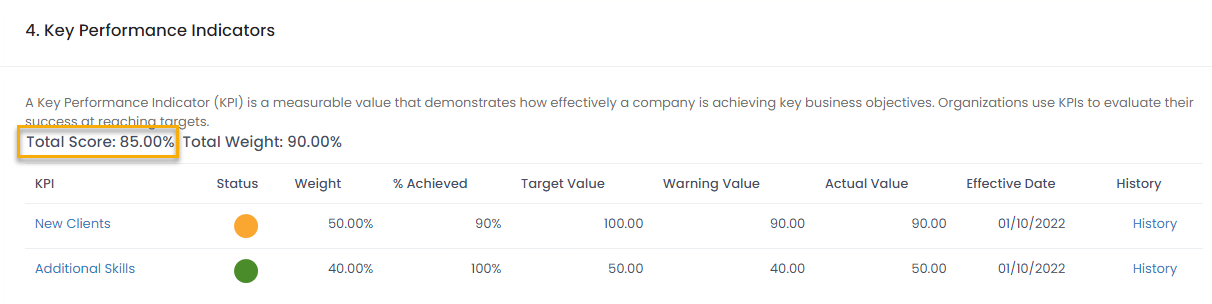The total score is calculated on the basis of the following criteria:

• Competencies. During the competency assessment, each competency is assigned some score based on the Competency Levels (Settings > Settings and Configuration > Performance > Competency).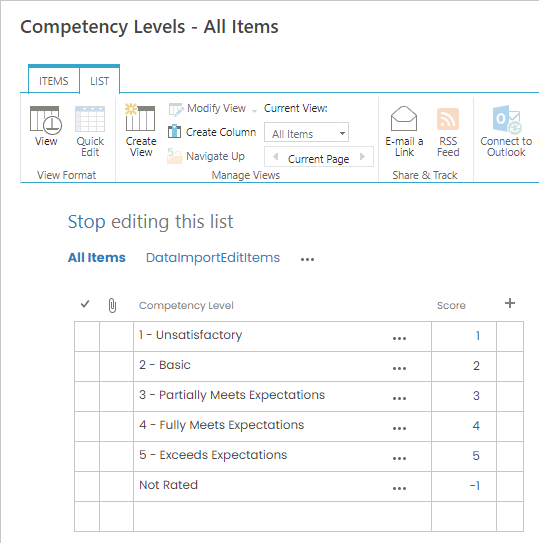The maximum level score is considered to be 100% and in relation to it, the percent for the assigned score is calculated. For example, the maximum score is 5 (Exceeds expectations) and the competencies were assessed as 3 (Partially Meets Expectations) and 4 (Fully Meets Expectations). The total score will be 3.5, that is 70%.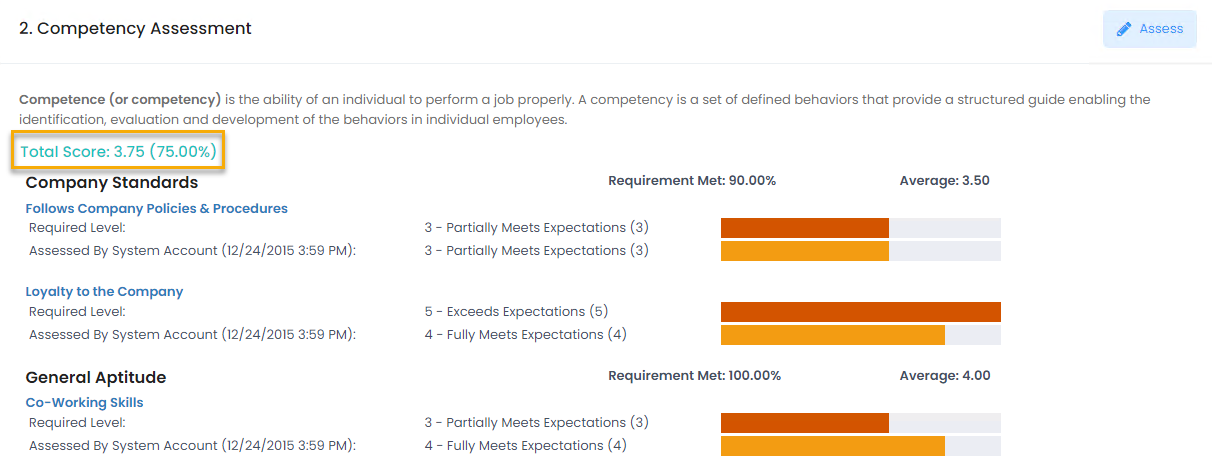• Goals and KPIs. Each goal and KPI have weight and percent complete (% Achieved for KPIs).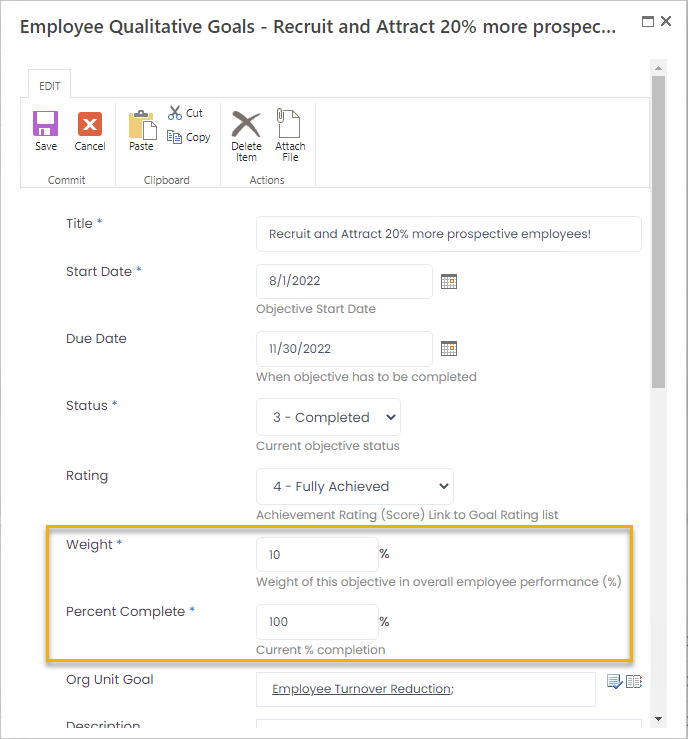The percent compete for the qualitative goals is specified manually. For the quantitative ones, it is calculated as the actual result compared to the target result. The Max Result and fields also influence the calculation if specified. The Max Result acts as .

The formulas for calculating the percent complete for quantitative goals are the following:
• For quantitative goals with Best Value set to More percent complete is calculated as follows:
• If 'Actual Result' is empty, then 'Percent Complete' = 0
• If 'Threshold' is not equal to 0 and also 'Actual Result' <= 'Threshold', then 'Percent Complete' = 0
• If ('Actual Result' <= 'Target Result' or 'Actual Result' <= 'Max Result') and 'Target Result' > 0, then 'Percent Complete' = 'Actual Result' / 'Target Result'
• If ('Actual Result' <= 'Target Result' or 'Actual Result' <= 'Max Result') and 'Target Result' <= 0, then 'Percent Complete' = 0
• If ('Actual Result' > 'Target Result' or 'Actual Result' > 'Max Result') and 'Target Result' > 0 and 'Max Result' > 0, then 'Percent Complete' = 'Max Result' / 'Target Result'
• If ('Actual Result' > 'Target Result' or 'Actual Result' > 'Max Result') and 'Target Result' > 0 and 'Max Result' <= 0, then 'Percent Complete' = 'Actual Result' / 'Target Result'
• If ('Actual Result' > 'Target Result' or 'Actual Result' > 'Max Result') and 'Target Result' <= 0 then 'Percent Complete' = 0
• For quantitative goals with Best Value set to Less percent complete is calculated as follows:
• If 'Threshold' = 0 and 'Actual Result' <= 'Target Result', then 'Percent Complete' = 1
• If 'Threshold' = 0 and 'Actual Result' > 'Target Result', then 'Percent Complete' = 0
• If 'Threshold' = 'Target Result', then 'Percent Complete' = 0
• If 'Threshold' is not equal to 0 and 'Threshold' is not equal to 'Target Result' and 'Actual Result' >= 'Threshold', then 'Percent Complete' = 0
• If 'Threshold' is not equal to 0 and 'Threshold' is not equal to 'Target Result' and 'Actual Result' >= 'Max Result', then 'Percent Complete' = ('Threshold' - 'Actual Result') / ('Threshold' - 'Target Result')
• If 'Threshold' is not equal to 0 and 'Threshold' is not equal to 'Target Result' and 'Actual Result' < 'Threshold' and 'Actual Result' < 'Max Result', then 'Percent Complete' = ('Threshold' - 'Max Result') / ('Threshold' - 'Target Result')

For KPIs, the result is calculated on the basis of the target and actual values according to the following formula:

• For KPIs with Best Value set to More:
•  If 'Target Value' > 0, then 'Percent Achieved' = ('Actual Value' / 'Target Value') * 100
• For KPIs with Best Value set to Less:
• If 'Target Value' is not equal to 0 and 'Actual Value' <= 'Target Value', then 'Percent Achieved' = 100
• If 'Target Value' is not equal to 0 and 'Actual Value' > 0, then 'Percent Achieved' = (TargetValue / ActualValue) * 100
• If 'Target Value' = 0 and 'Actual Value' = 0, then 'Percent Achieved' = 100
• If 'Target Value' = 0 and 'Actual Value' >= 'Warning Value', then 'Percent Achieved' = 0
• If 'Target Value' = 0 and 'Actual Value' < 'Warning Value', then 'Percent Achieved' = (1 - 'Actual Value' / 'Warning Value') * 100

On the basis of the percent complete/percent achieved and weight (must be 100% for all goals and for all KPIs) of the goals/KPIs, the total score is calculated as follows:

For goals:

'Goal Score' = 'Percent Complete' * 'Weight' / 100

'Total Score' = Sum of all goal scores

For example, the employee has 2 goals for the review period. One goal has weight of 75% and is completed for 100%, another goal has weight of 25% and is completed for 50%. The Total Score will be 87.5% (Total Score = (100*75/100) + (50*25/100) = 87.5%)

For KPIs:

'KPI Score' = 'Percent Achieved' * 'Weight' / 100

'Total Score' = Sum of all KPI scores

Note
If the rating is specified for a goal in the Rating field, it will be included in the total score calculation instead of Percent Complete. In this case, the score of the selected rating is compared to maximum rating to define the score percent achieved.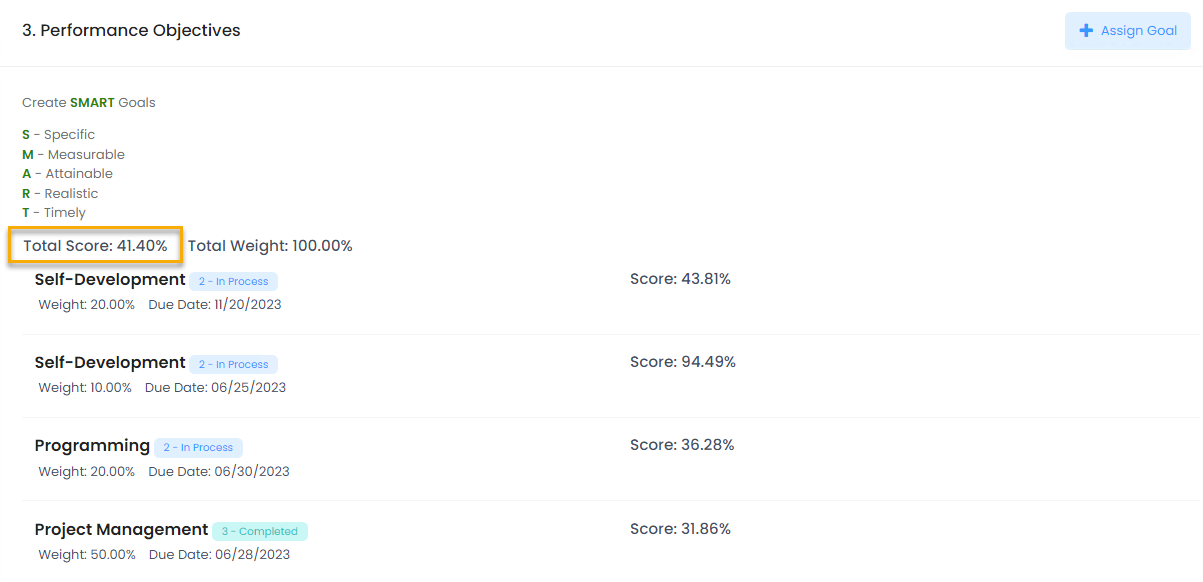The Calculated Score is calculated based total scores of all the three sections (Competencies, Goals and KPIs) according to the following formula: (Competency Total Score  * Competency section weight) + (Goals Total Score * Goals section weight) + (KPI Total Score * KPI section weight). By default, all the sections have the same weight and equally influence the calculated score. However, the users can assign weight to each section so that calculated score depends more on one sections and less on the others. For example, the weights can be defined as follows: Competencies - 50%, Goals - 25% and KPIs - 25%. For more details on setting up the section weights, refer to the Weighted Sections for Calculated Score topic.

Note
The calculated scores (total scores) for the individual sections (Competencies, Goals and KPIs) are stored in the Performance Review list, the Competencies Score, Objectives Score and the KPIs Score fields, accordingly. The scores are recorded there after submitting the review form. These fields can be used for creating custom reports or views.